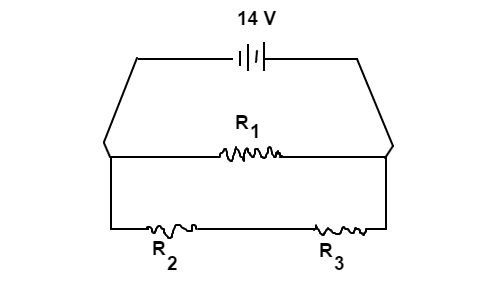# Triple Resistor CircuitThe circuit design, shown above, consists at a $14 \text{V}$ battery and three resistors ($R$). All three resistors have resistances of $6 \text{Ω}$. Determine the current ($I$) of the circuit in $\text{amps}$.

Hint: $V=IR$

×